« Exercise 4.9.4 Exercise 4.9.6 »

Exercise 4.9.5

Given : Covering load gεπ=1.5 kN/m2, live load q=5.0 kN/m2, concrete quality C40/50.

Question : Perform static analysis of the slabs illustrated in the figure (shears forces, bending moments, deflections) using the finite element method with (a) global loading and (b) unfavourable loadings.

Solution:

Slabs are identical to those of the example of §4.6.4.

g=0.15x25.0+1.5=5.25 kN/m2

q=5.0 kN/m2

In ULS, the maximum design load is equal to:

pd=1.35g+1.50q=1.35x5.25+1.50x5.0=

=14.59 kN/m2

Software requires only characteristic loads of the structure to be inserted while the rest are obtained through internal calculations.

Due to both structure and loading being symmetric, results will also be symmetrical for both slabs.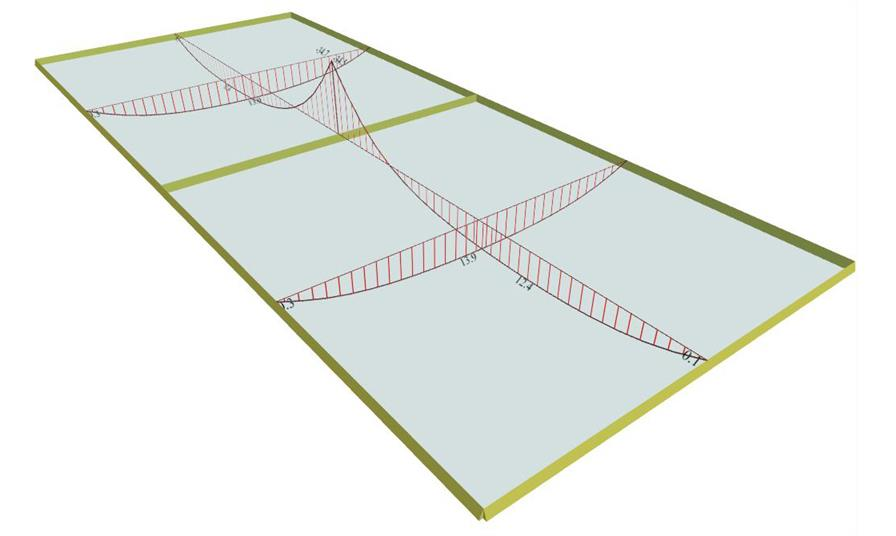Figure 4.9.5-2: Bending moment diagrams Figure 4.9.5-2: Bending moment diagrams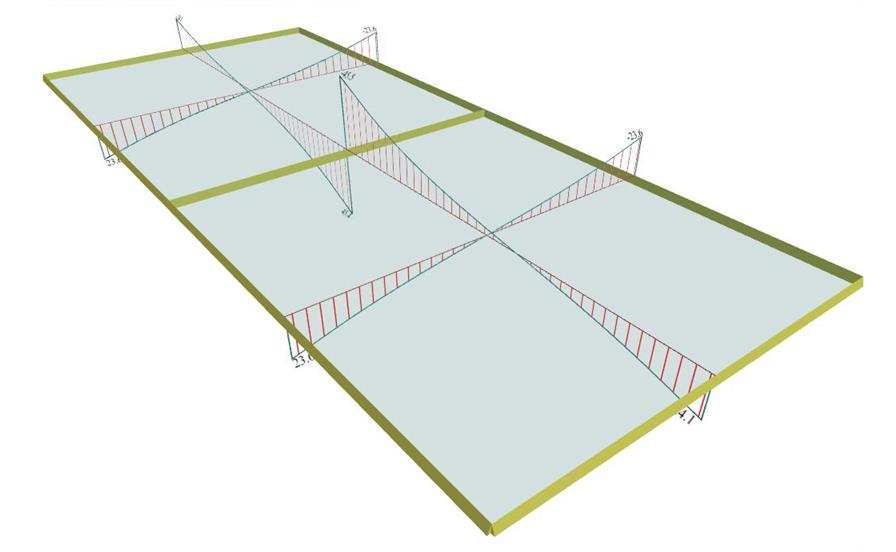Figure 4.9.5-3: Shear force diagrams Figure 4.9.5-3: Shear force diagrams Mx=13.9 kNm, My=12.4 kNm, Myerm=-34.6 kNm Vxr=23.6 kN, Vyr=24.1 kN, Vyerm=45.5 kN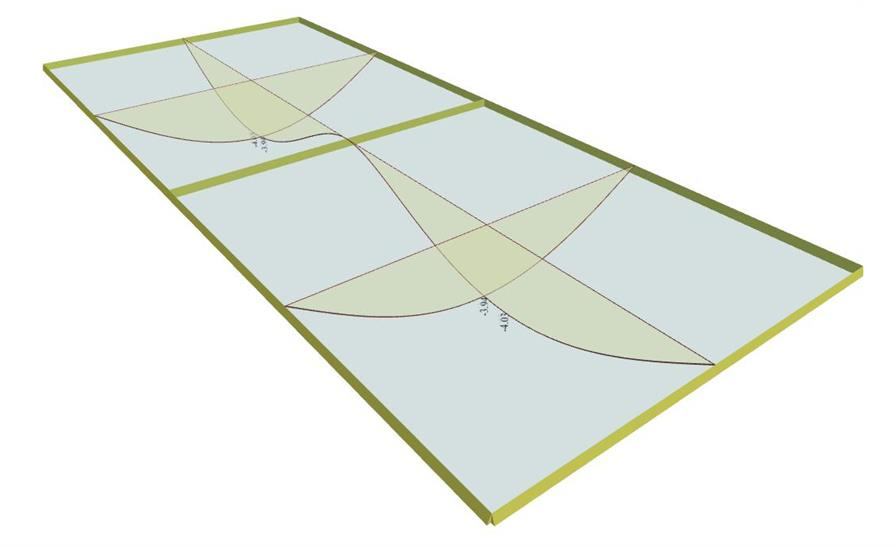Figure 4.9.5-4: Deflection diagrams Figure 4.9.5-4: Deflection diagrams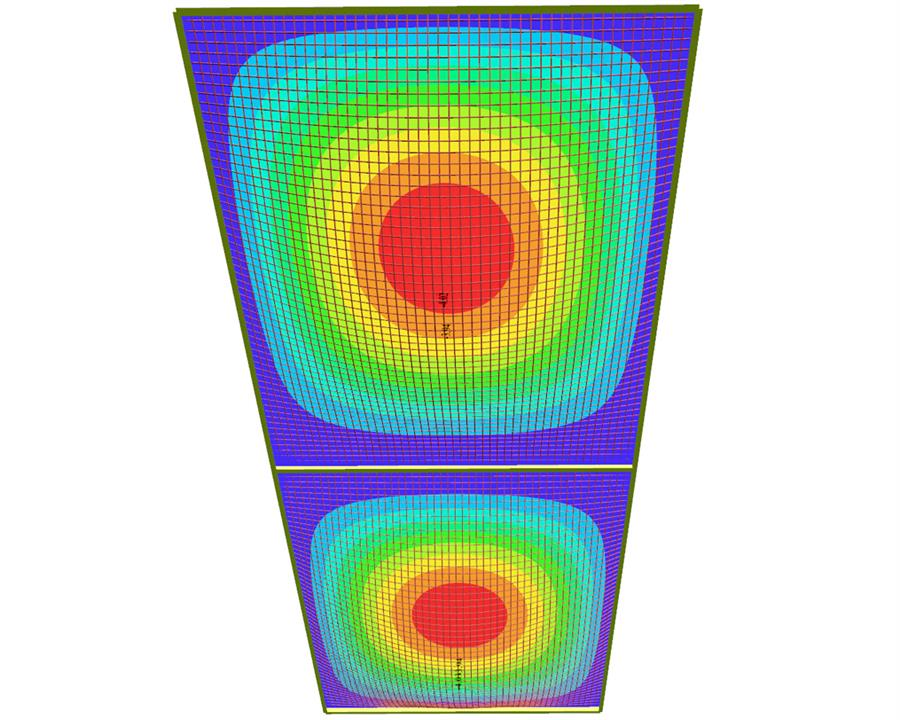Figure 4.9.5-5: Deflection diagrams and contours Figure 4.9.5-5: Deflection diagrams and contours ymax=4.03 mm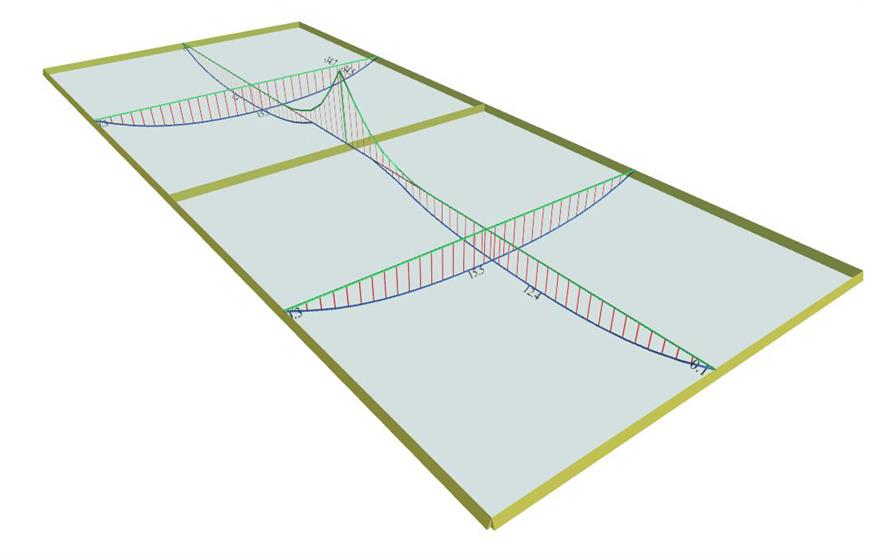Figure 4.9.5-6: Bending moment diagrams Figure 4.9.5-6: Bending moment diagrams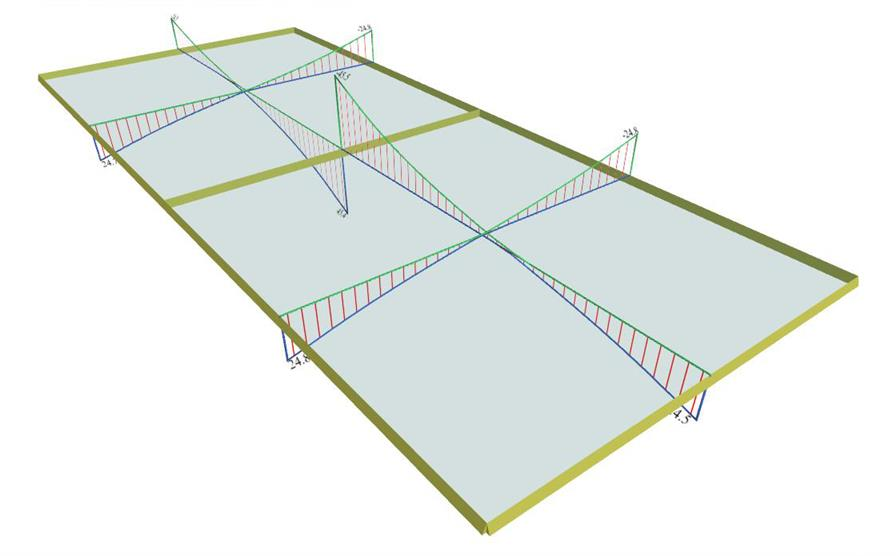Figure 4.9.5-7: Shear force diagrams Figure 4.9.5-7: Shear force diagrams Mx=15.5 kNm, My=12.4 kNm, Myerm=-34.6 kNm Vxr=24.8 kN, Vyr=24.5 kN, Vyerm=45.5 kN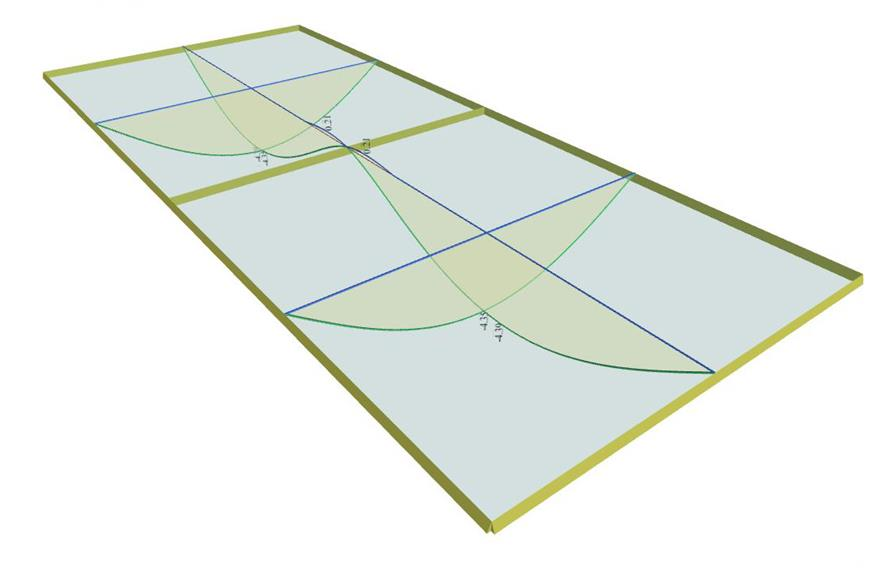Figure 4.9.5-8: Deflection diagrams Figure 4.9.5-8: Deflection diagrams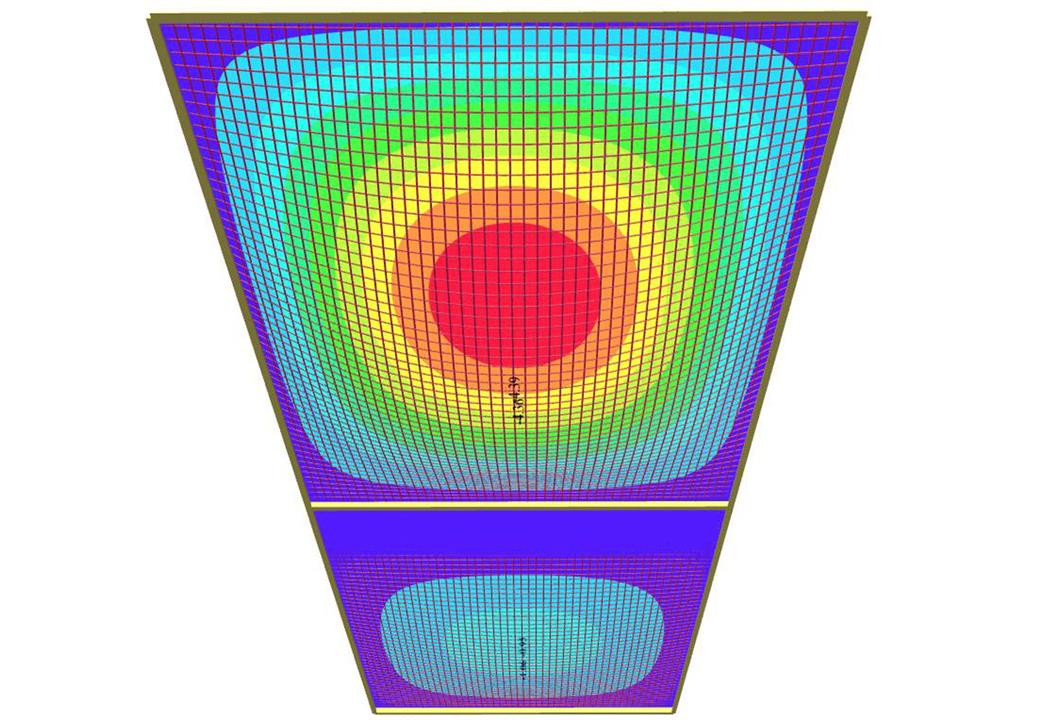Figure 4.9.5-9: Deflection diagrams and contours Figure 4.9.5-9: Deflection diagrams and contours ymax=4.39 mm [*]NoteSince in “Adverse” state analytical diagrams are not provided, in ‘Element Input’, live load and covering load of the 2nd slab are eliminated, thus approaching the necessary difference of (1-1/1.35)g=0.26g. In this case, the slabs loading is represented by the respective unfavourable loading on their spans.

Notes:

• The resulting span moments for unfavourable loadings are higher than those for global loading, only in the span along direction x.

• Support moment remains the same, because unfavourable and global loadings are identical.

« Exercise 4.9.4 Exercise 4.9.6 »